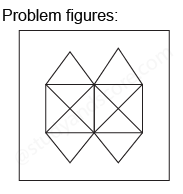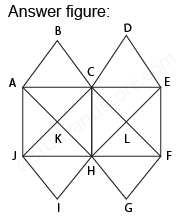## Practice Test Discussion

Q)

What is the minimum number of straight lines that is needed to construct the figure?• ExplanationAfter labelling the figure, we get the following,

• The horizontal lines are AE and JE (2)
• The vertical lines are AJ, CH and EF (3)
• The slanting lines are AG, BF, JD, IE, AB, DE, JI and FG (8)

Total number of straight lines in the figure are 2 + 3 + 8 = 13

Hence option B is correct.

- Share with your friends! -